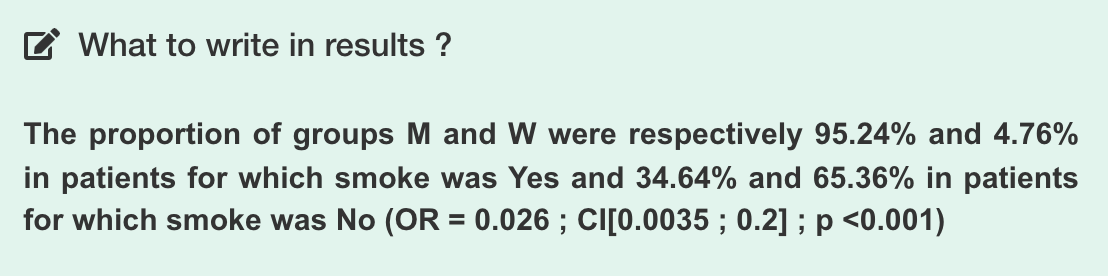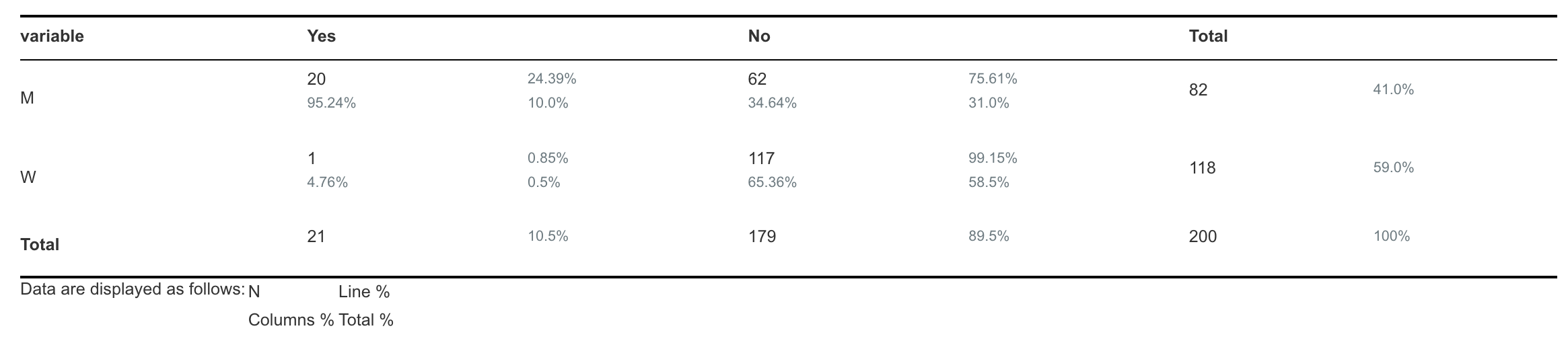# Calculate an Odds Ratio (OR)

Modified on Sun, 05 Dec 2021 at 10:34 AM

# What is an Odds-Ratio?

An odds ratio (OR) is a statistic that quantifies the strength of the association between two events, A and B.

Odds-Ratio is not the same as relative risk (RR). Relative risk is calculated on the whole population.

However, in clinical studies, an odds-ratio is generally preferred because data for the entire population is not available.

# How to calculate an Odds-Ratio?

To calculate an OR, you need 2 variables with 2 modalities for each variable. It could be 2 Yes-no variables or 2 List variables with 2 possible values.

For example, it could be Smoker (Yes-no) vs Gender (List with 2 possible values).

1. In the "Statistics" menu, click on "Test variables"
2. Pick the first variable (for example "Smoker")
3. Pick the second variable (for example "Gender")
4. Open the panel "Find an association between ..."
5. The odds-ratio and its confidence interval are displayed in the "What to write in results" boxThe calculated OR is 0.026 with a confidence interval ranging from 0.0035 to 0.2

# The OR is not calculated

There are 2 main reasons why an OR would not be calculated:

1. One of the cells of the contingency table is equal to 0
2. A List variable with more than 2 values

## One of the cells of the contingency table is equal to 0

The contingency table is a table generated from the distribution of the 2 variables. It represents the frequency of each possible combination of each variable. In the example below, it counts how many patients are both "Women" and "Diabetics" (n=1), both "Women" and "Non-diabetics" (n=117), etc...The Odds-Ratio can not be calculated if at least one of the cells of the table is equal to 0.

## A List variable with more than 2 values

An OR can only be calculated if the contingency table has 4 cells. If one of your variable has more than 2 possible values (modalities), then an OR will not be computed.

However, you can group some values together to obtain only 2 values. For example, if you have a variable "Smoker" with 3 modalities "Never smoker", "Former smoker" and "Active smoker", you could group together "Never smoker" and "Former smoker". See the following link for more information: How to group values (modalities) of a List variable?